# Derivatives Example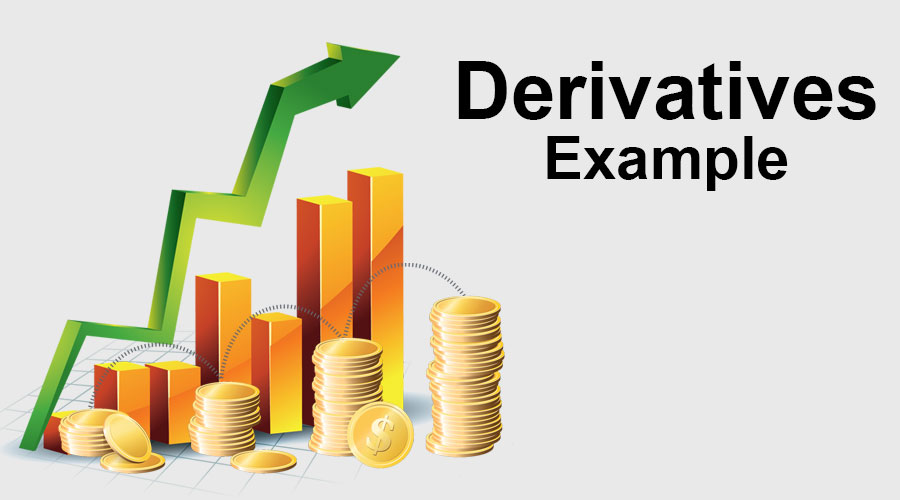## Introduction to Derivatives Example

The following derivative example provides an overview of the most prevalent kinds of derivative instruments. A derivative is a financial security whose value is derived from an underlying asset. Underlying assets can be equity, index, foreign exchange, commodity, or any other assets. So from the above definition, it is clear that derivative products do not have their own value, its value is decided by any particular underlying assets. The main participant in derivative markets are hedgers, speculators, and arbitragers. Below examples of a derivative illustrate of the most common derivatives. It is impossible to provide all types of derivative examples, since there thousands of such derivatives, and which vary in every situation.

### Examples of Derivatives (With Excel Template)

Let’s try to understand derivatives through the below examples.

You can download this Derivatives Example Excel Template here – Derivatives Example Excel Template

#### #1 Derivatives Example – Futures Contract

ABC Co. is a delivery company whose expenses are tied to fuel prices. ABC Co. anticipated that they use 90,000 gallons of gasoline per month. It is currently, July 1st and the company wants to hedge its next 3 months of fuel costs using the RBOB Gasoline future contracts. Information on these contracts is as follows.

• Each contract is for 42,000 gallons.
• Contracts expire at the end of the prior month. For example, if we have to buy the August contract that will expire at the end of July.
• The initial margin is \$11,475 and the maintenance margin is \$8,500.

Given,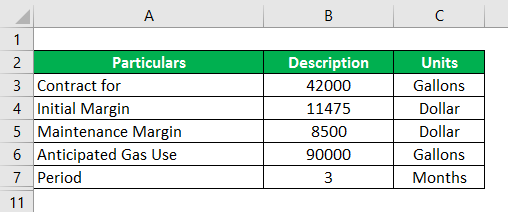Question-1 – Should ABC Co. Buy (Long) or Sell (Short) the Future to Initiate its Position.

Solution:

ABC Co. exposure is to the gas price if the gas price goes up, its expenses will go up, due to expenses profit will go down. So if an ABC Co want to hedge that risk exposure and protect its profit, they need a situation where future position going to increase in value when gas prices go up. So if a company go for long Contract buy gasoline futures so that the company will make a profit on that futures when gas goes up so this will offset with natural risk exposure. So the ABC Co. hedge position here is to go long (buy) the contract.

Question 2 – How many contracts should ABC Co. use?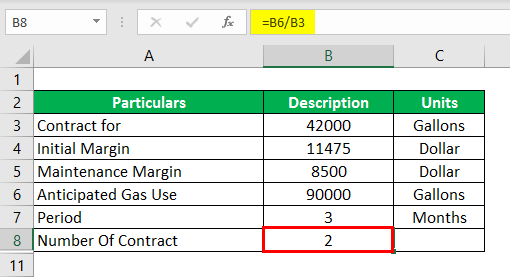Solution:

ABC Co. uses 90,000 Gallons of Gas every Month and each Contract was for 42,000 Gallons.

• Number of Contracts = Anticipated Gas Use / Contract for
• Number of Contracts = 90000/42000
• Number of Contracts = 2

For Two Contract:

•  One contract = 42,000
• Two contracts = 2 × 42,000
• Two contracts = 84,000 Gallons of Gas.

So how many should be used the answer is 2.

Question 3 -What is ABC Co. initial cash flow?

Initial Cash Flow or Margine is Calculated as :

Solution: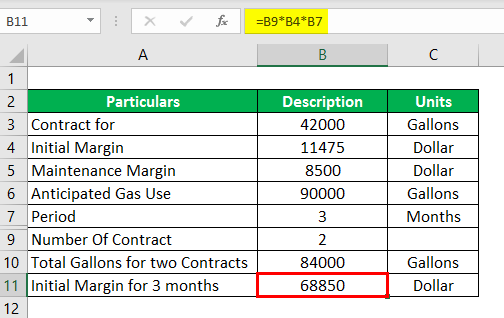• So here number of Contract = 2
• Initial  Cash Flow/Margin = \$11,475
•  = 2 × \$11,475
• = \$22,950 per month

So for 3 month

•  Initial Cash Flow/ Margin For 3 month = \$22,950 × 3
•  Initial Cash Flow /Margin For 3 month = \$68,850

So initially ABC Co. has to put \$68,850 into its margin accounts in order to establish its position which will give company two contacts for next 3 month.

Question 4 – The price of gasoline for the August future is \$2.8974, September future is \$2.8798 and the October future is \$2.7658 and which closed at August \$2.6813, September \$2.4140 and October \$2.0999 How much did ABC Co. lose on a futures contract?

Solution:

Loss = (Closing Price – Opening Price) × Total Gasoline

Loss is Calculated as Below: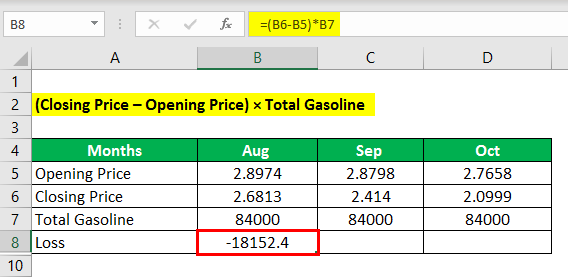• Loss = (2.6813-2.8974) * 84000
• Loss = -18152.4

Similarly for all,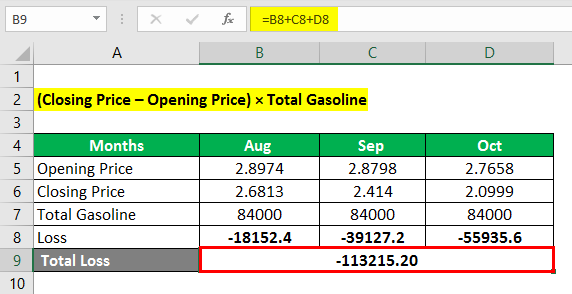• Total Loss = Loss In (Aug) + (Sep) + (Oct)
• Total Loss = -18152.4 + -39127.2 + -55935.6
• Total Loss = -113215.20

So Total loss on Futures Contracts \$-113215.20

#### Derivatives Example#2 – Long Futures

On 1st March an Indian importer enters a contract to import 1,000 bales of cotton with payments to be made in dollars on 1st September. The price of one bale of cotton was fixed at USD 50 per bale. The present exchange rate is 1 USD = 69.35 INR. The importer has the risk of paying the more if USD strengthens. The dollar strengthens in the coming months and on the 1st of September, the exchange rate climbs to 1 USD = 72.35 INR. Let us look at the following two scenarios.

Now what has happened here that Importer has to pay more due to rate difference i.e. 72.35 INR – 69.35 INR = 3 INR

So 1000×50×3 = INR 1, 50,000 extra amount to pay.

Given,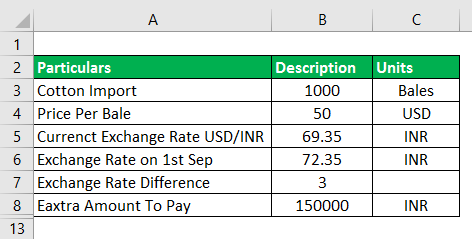Case -1:- When the Importer has not hedged his position.

Solution:

The Total Payment made in USD as on 1st March = No. Of cotton bales × per unit price

The Total Payment made in USD as on 1st March is as calculated below: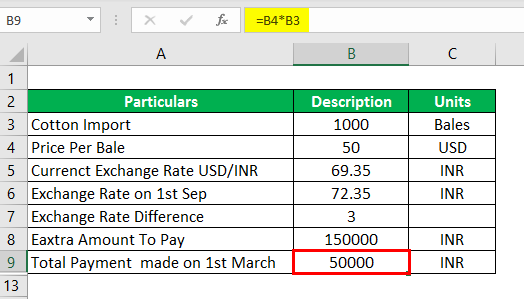• The Total Payment Made on 1st March = 50×1000
• The Total Payment Made on 1st March = \$50,000

Amount of INR required to make a payment on 1st March

Amount of INR required to make a payment on 1st March are as calculated below: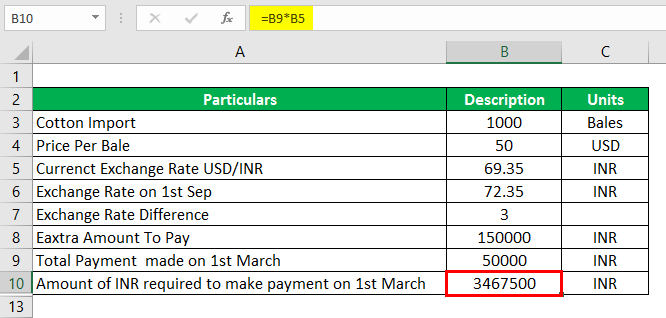• Amount of INR Required to make a Payment on 1st March = \$50,000 × 69.35
• Amount of INR Required to make a Payment on 1st March = 34, 67,500 INR

Amount of INR Required to Make a Payment on 1st Sep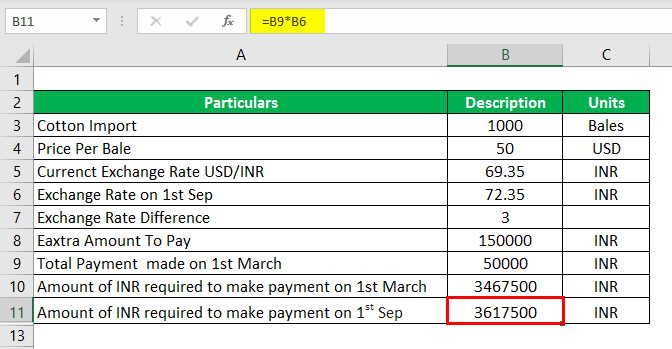• Amount of INR Required to make a Payment on 1st Sep = \$50,000 × 72.35
• Amount of INR Required to make a Payment on 1st Sep = 36, 17,500 INR

Total Loss Suffered due to an Increase in the Exchange Rate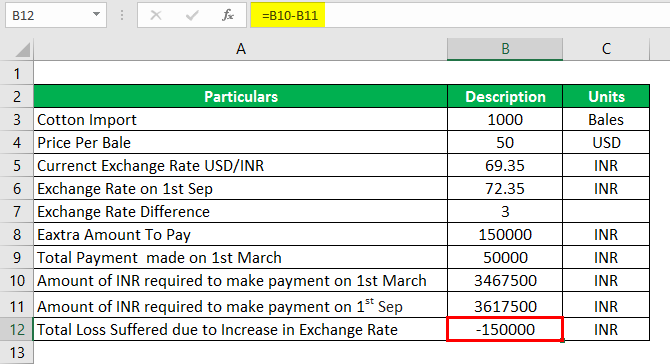• Total Loss Suffered due to an Increase in the Exchange Rate = 34, 67,500.00 – 36, 17,500.00
• Total Loss Suffered due to an Increase in the Exchange Rate = -1, 50,000 INR

Conclusion– The Importer has to pay an extra 1, 50,000.00 INR on 1st September due to an increase in the exchange rate thus incurs a loss compared to his payment obligation as on 1st March.

Case -2:- The Importer decided to hedge his position by going in the currency futures market. The importer expected that USD will strengthen and he decided to USD-INR contract to hedge his position.

Solution:

No of USD –INR Contracts

No of USD –INR Contracts are calculated as: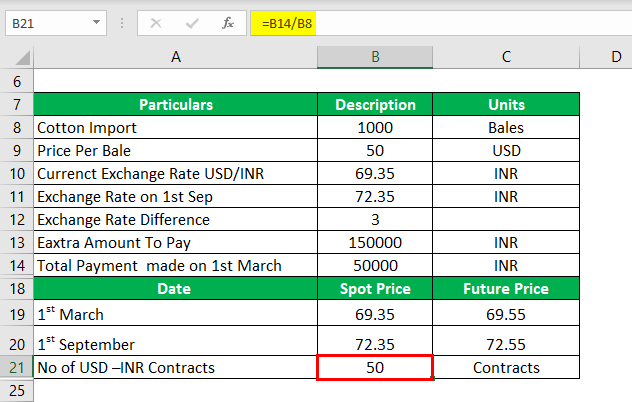• No of USD –INR Contracts = Amount to Pay/1000 (Lot size for 1USD-INR Contract)
• No of USD –INR Contracts = 50,000/1000
• No of USD –INR Contracts = 50 Contracts

Total Amount Incurred on buying a Currency Futures Contract

Total Amount Incurred on buying a Currency Futures Contract is calculated as :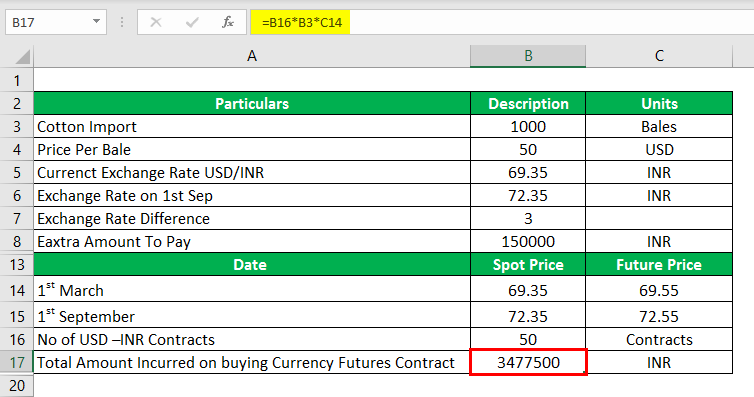• Total Amount Incurred on buying a Currency Futures Contract = 50 × 1000 × 69.55
• Total Amount Incurred on buying a Currency Futures Contract = 34, 77,500 INR

Proceeds from the Sale of Future Contract

Proceeds from the Sale of Future Contract is Calculated as :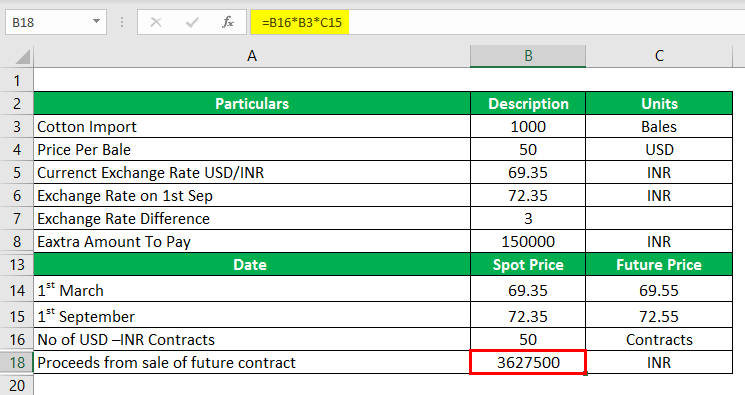On 1st September the Exchange Rate moves to 72.35 and the Future Price Moves to 72.55

• Proceeds from the Sale of Future Contract = 50 × 1000 × 72.55
• Proceeds from the Sale of Future Contract = 36, 27,500 INR

Profit on Sale of Future

Profit on Sale of Future is calculated as :

Profit on Sale of Future = Sale of Future Contract – Purchase of Future Contract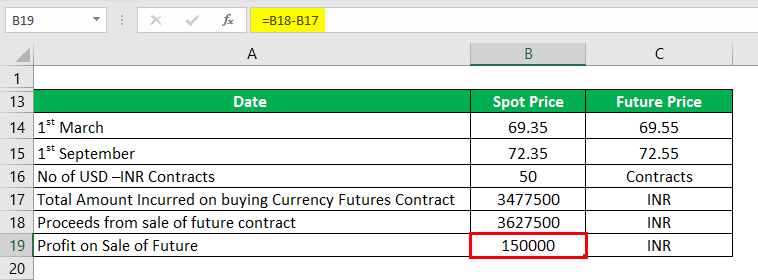• Profit on Sale of Future = 36, 27,500.00 – 34, 77,500.00
• Profit on Sale of Future = 1, 50,000 INR

Conclusion: The Importer has effectively hedged his loss by entering in the future contracts and thereby null and void his loss because of adverse movement in the exchange rate.

#### Derivatives Example#3 – Stock Index Futures

John owns a stock portfolio and detail related to the portfolio as mentioned below.

• Value of portfolio: V =\$95 Million (Spot Price)
• Beta of portfolio: β =0.90

Future contract S&P Future price:

• f= 1,513.40
• S&

The post Derivatives Example appeared first on EDUCBA.

This post first appeared on Best Online Training & Video Courses | EduCBA, please read the originial post: here